Definitions
Name the Shortcut
All My Angles
Missing Criteria
Shortcut Criteria
100

What does SAS mean?

Side-Angle-Side: If two pairs of sides of two triangles are equal in length, and the included angles are equal in measurement, then the triangles are congruent.

100

If three sides of one triangle are congruent to three sides of another triangle, and the triangles are congruent.

SSS

100

Each triangle has how many interior angles?

3

100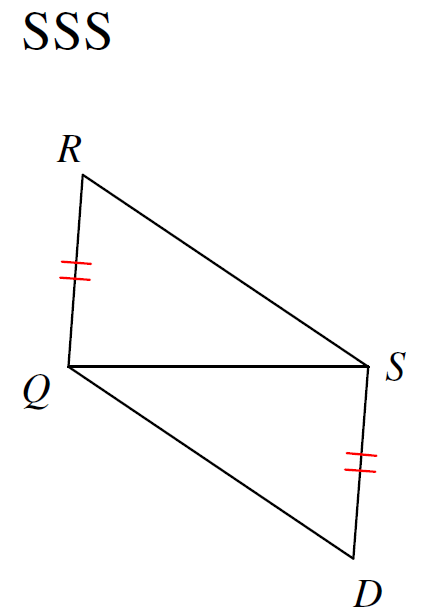RS and QD need to be congruent in order to prove these triangles congruent using SSS.

100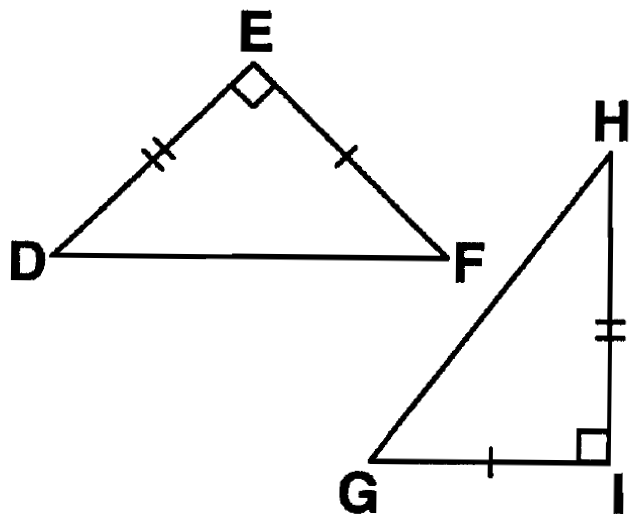SAS

200

What does SSS mean?

Side-Side-Side: If three pairs of corresponding sides of two triangles are equal in length, then the triangles are congruent.

200

If two sides and the included angle of one triangle are congruent to the corresponding parts of another triangle, the triangles are congruent.

SAS

200

What is the sum of all three interior angles in a triangle?

180 degrees

200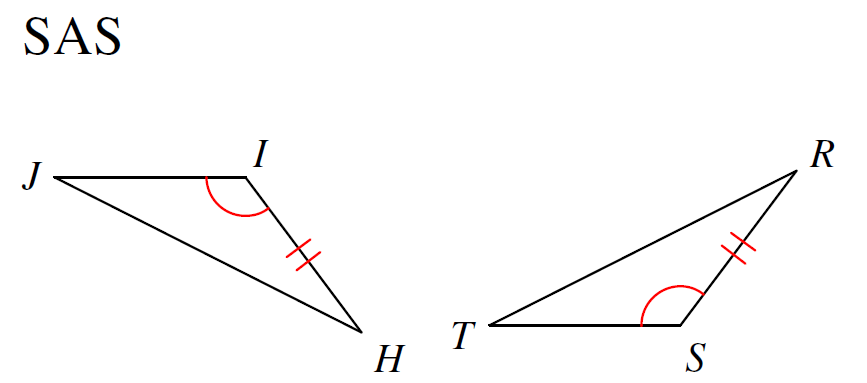JI and TS need to be congruent in order to prove that the triangles are congruent using SAS.

200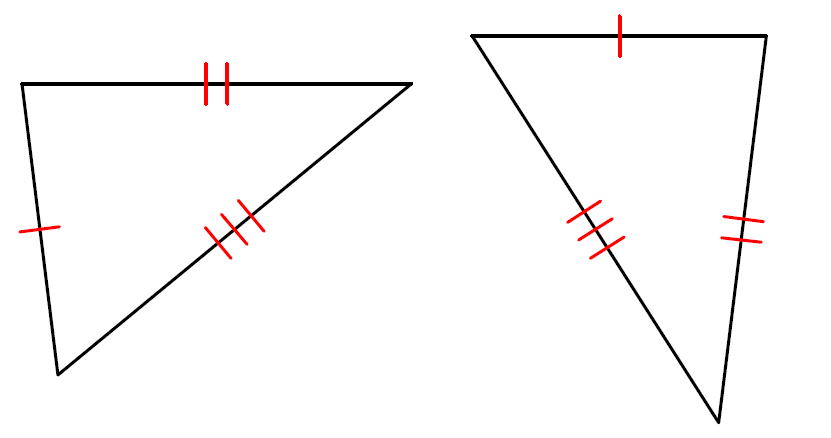SSS

300

What does ASA mean?

Angle-Side-Angle: If two pairs of corresponding angles of two triangles are equal in length and the included sides are equal, then the triangles are congruent.

300

If two angles and the included side of one triangle are congruent to the corresponding parts of another triangle, the triangles are congruent.

ASA

300

What kind of angle is notated by a box/square?

A right angle

300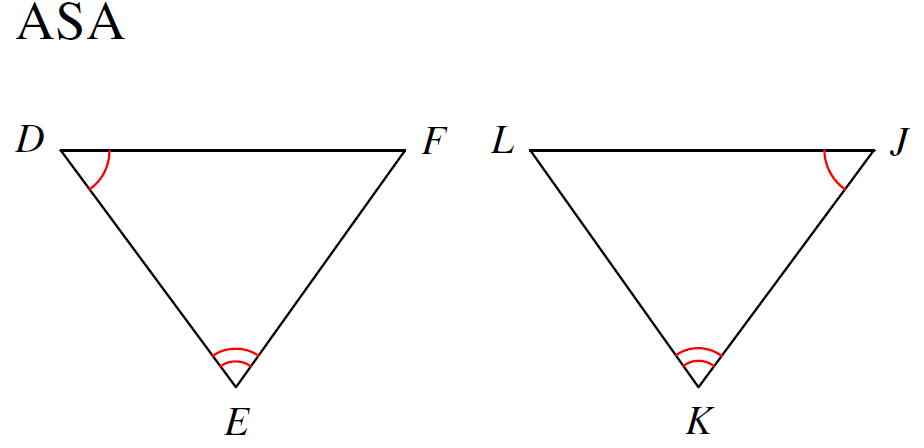Missing DE congruent to KJ

300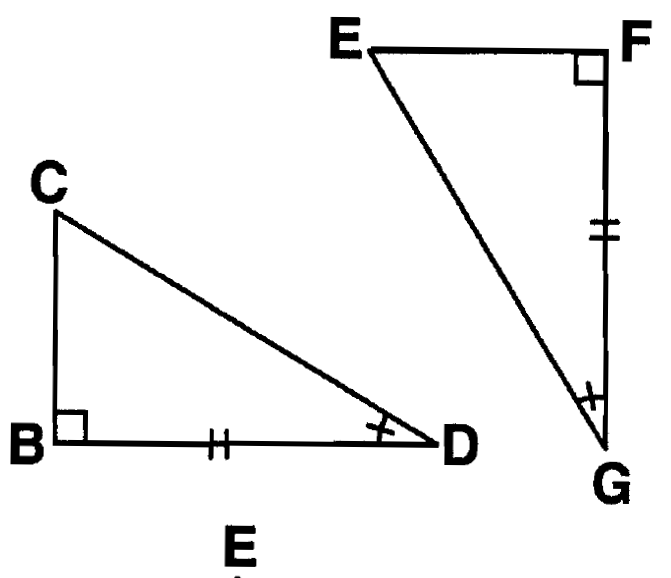ASA

400

What does AAS mean?

Angle-Angle-Side: If two pairs of corresponding angles of two triangles are equal in measurement, and a pair of corresponding sides are equal in length, then the triangles are congruent.

400

If two angles and the non-included side of one triangle are congruent to the corresponding parts of another triangle, the triangles are congruent.

AAS

400

Even though a triangle may have three congruent angles, this shortcut does not guarantee to show those two triangles will be congruent.

AAA

400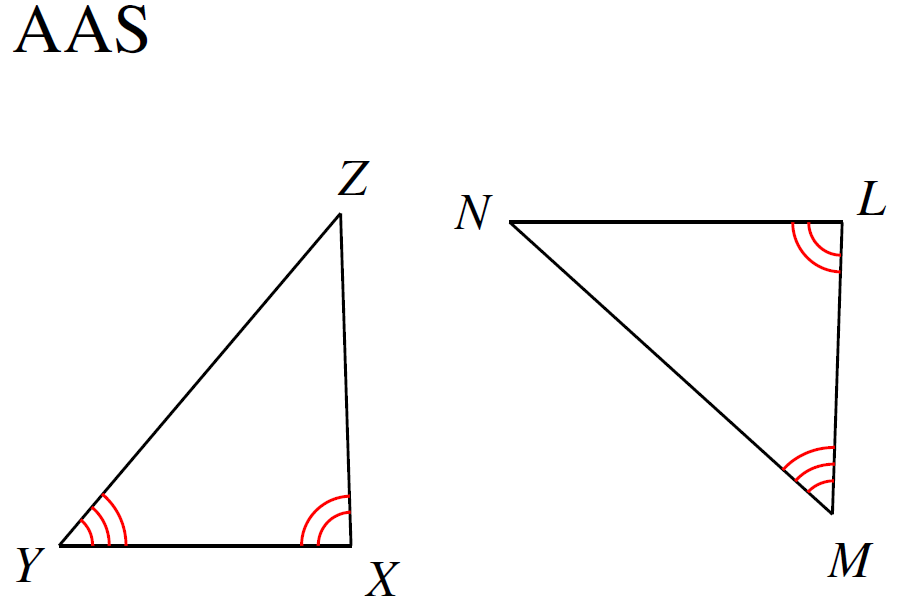Missing YZ congruent to MN or XZ congruent to LN

400SSS

500

State the 4 shortcuts that DO work to show two triangles are congruent.

SSS, SAS, ASA, & SAA

500

This shortcut fails to guarantee congruent triangles because you can create TWO different triangles instead of one.

SSA

500Intersecting lines create these kind of angles.

Vertical angles

500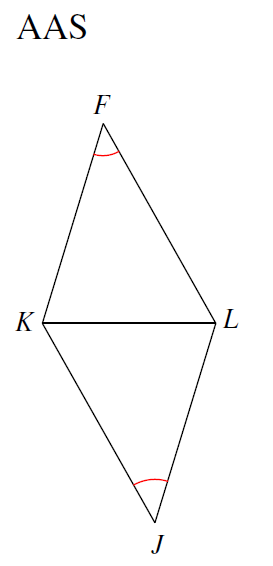Missing angle FKL congruent to angle JKL or angle FLK congruent to angle JKL

500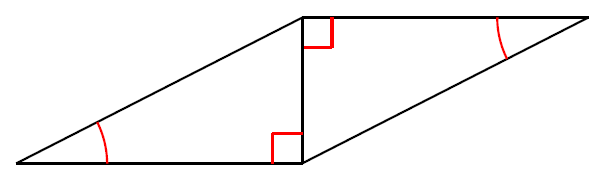SAA

Click to zoom